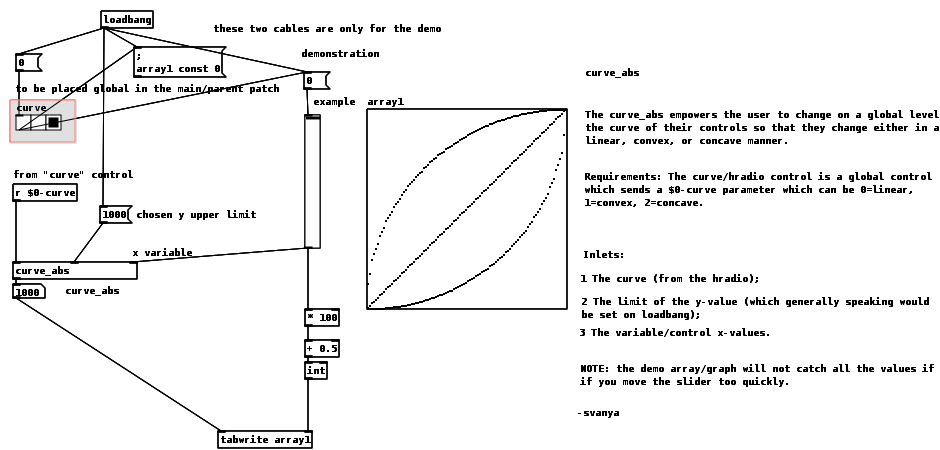The curve_abs empowers the user to change on a global level the curve of their values so that they change either in a linear, convex, or concave manner (see image, curve_abs-help.pd.png, below for reference).

Requirements: The curve/hradio control is a global control which sends a \$0-curve parameter which can be 0=linear, 1=convex, 2=concave.

Inlets:

1 The curve (from the hradio);

2 The limit of the y-value (which generally speaking would be set on loadbang);

3 The variable/control x-values.

Demo included in the curve_abs-help.pd file.curve_abs-help.pd
curve_abs.pd

Ciao for now. Peace

-svanya